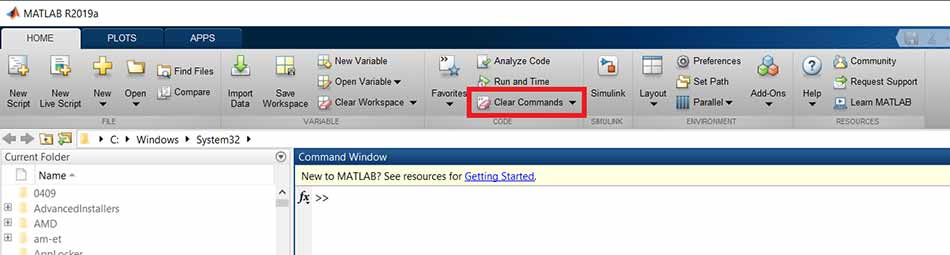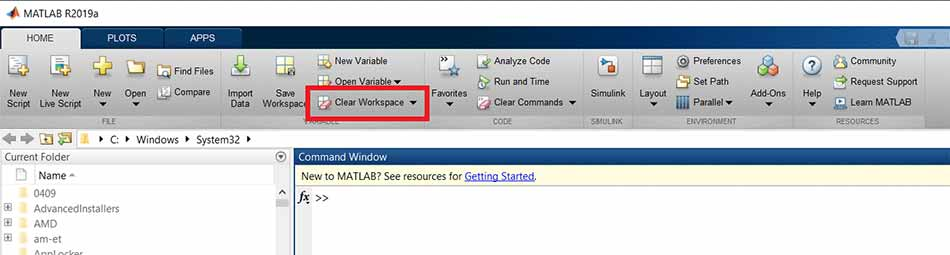## Important constants in MATLAB tricks

MATLAB has a set of special numbers and operators. In the following, we have introduced some frequently used cases.

## Pi number in MATLAB

The number π (pi or pi) is one of the most important constants in MATLAB. If you want to do trigonometric operations in MATLAB, you will probably need this number. In MATLAB you do not need to define a variable and set its value to π, ie 3.14. Why? Because MATLAB has defined this number for you. Wherever you need the number π, just follow the instructions below:

```> pi
ans =
3.1416```

That is, it is enough to write pi in your code and that’s it! Only four decimal places for π are shown here. But in fact the decimal number is very high. The number π also has many digits in MATLAB, but only 4 decimal places are displayed. By default, MATLAB shows outputs up to 4 decimal places. However, in this session, we will show you how to see more decimal places.

You can treat pi like any other variable. That is, you can multiply, divide, add, or subtract pi into other numbers. Or give it to functions as input.

Exercise 1: Create a variable called angle with a value of π / 2.

> angle = pi / 2;

## Neper number in MATLAB

The number neper (e) is another important constant. However, like pi, no specific variable is defined for this number. But we can access this number using the expression exp (1). We haven’t talked about functions yet. So please do not be curious about exp (1)! You will find out more in future sessions. So to have a neper number, it is enough to write:

```>> exp ( 1 )
ans =
2.7183```

## eps in MATLAB

One of the other variables you need in MATLAB is eps. eps stands for epsilon. You probably all know what this symbol is used for. The eps symbol is often used to represent very, very small numbers that are close to zero. This number is a fixed number in MATLAB. The eps value in MATLAB is equal to 2.2204e-16. Like pi and neper, eps can be divided by multiplication. Wherever you need eps, just follow the instructions below:

```>> eps
ans =
2.2204e-16```

## inf in MATLAB

Another widely used constant in MATLAB is inf. inf stands for infinity. To show infinity in mathematics we use the symbol .. The inf constant is equal to the symbol.. Wherever you need ∞ in MATLAB, just follow the instructions below:

```> inf
ans =
Inf```

Exercise 2: Divide the number one by zero and see the result.

The answer is inf. Although the division of a number into zero is not defined in mathematics, MATLAB knows the result of this division to be inf!

## nan in MATLAB

Another constant we may encounter is NaN. NaN stands for Not a Number. NaN is equivalent to the term “undefined” in mathematics. If you remember, we would say that the result of dividing zero by zero or infinity by infinity is the result of infinity. You may want to define a variable that is NaN, in which case you should write:

```> NaN
ans =
NaN```

By writing nan, we reach the same result:

```> nan
ans =
NaN```

Exercise 3: Divide the number zero by zero and see the result.

The result is NaN.

Exercise 4: Divide inf by inf and see the result.

Again, the result is NaN.

## Imaginary numbers in MATLAB

The use of imaginary numbers is one of the most important parts of the MATLAB tricks session. If you have worked with complex numbers, you are familiar with i and j. In imaginary numbers, i or j are equivalent to the square root of the number -1. In MATLAB, you can easily access these variables by typing i or j. Let’s practice. First, just write i to see what the result will be:

```> i
ans =
0.0000 + 1.0000i```

You see, MATLAB realized that you mean an imaginary number. Print the value 1i + 0.

Let’s multiply the value of i by 2:

```> i ^ 2
ans =
-1```

As you can see, the value of i is twice the power of -1.

Note that if you have already defined a variable called i or j, MATLAB will no longer recognize i or j as an imaginary number. For example, in the following code, we have already defined a variable called i with a value of 5:

```>> i = 5;
>> i
i =
5```

As you can see, MATLAB has set the value of i to 5. Because we have already defined a variable called i whose value is 5. You might think that if we want to use i to define imaginary numbers, we must first delete the variable i. But we have another suggestion. That means it has MATLAB! MATLAB says that if you write i in the form 1i, I consider i an imaginary number.

```>> i = 5;
>> 1i
ans =
0.0000 + 1.0000i
>> i =
5```

You can see that although we set i to 5, MATLAB does not care about it at all and considers 1i to be an imaginary number. What to do to define an imaginary number in MATLAB? For example, to define an imaginary variable equal to 3i + 8, it is enough to write:

```>> 3i + 8
ans =
8.0000 + 3.0000i```

Note that you can also put a multiplication sign between the number and i. But we recommend that you do not do this. Because if you have already defined another variable called i, MATLAB will consider it. For example, to define the same number 3i + 8, if we write this time we will have i * 3 + 8:

```>> 3 * i + 8
ans =
23```

You can see that MATLAB has set the value of i to 5! Because we used to set the value of i to 5. So in defining imaginary numbers, it is better to avoid the multiplication sign!

Exercise 5: Define a variable called x whose value is 4 + 9j.

`>> 9j + 4`
``` ans =
4.0000 + 9.0000j```

## Clear the command window with MATLAB tricks

After writing various exercise commands in the command window, you may not mind cleaning this window! To clean the Command Window you can use the clc command in MATLAB. In addition to clc, the command window can be deleted with just one click. Just click on the icon shown in the image below:clear-command-MATLAB

Clear the Command Window using the clc command.

`>> clc`

Note Running the clc command will only delete the command window entries. And this command will not delete variables in the workspace.

## Delete workspace in MATLAB

You may want to delete all workspace variables in MATLAB for any reason. For example, the workspace may be too crowded to define different variables. Or you want to start solving a new problem immediately after solving a problem and no longer need the variables of the previous problem. You can delete all variables from your workspace by typing clear in the Command Window.

Note If you do not save the variables and then delete the workspace, you will no longer have access to your variables. You may not realize the importance of variables now, but imagine that you have written a program and after a few days of execution, the output has been obtained. Then losing variables (including output response) will waste your days running!

In addition to the clear command, an icon is provided to clear the workspace. You can delete the workspace by clicking on the icon shown in the image below:clear workspace MATLAB

Note Most MATLAB professionals use the clear and clc commands instead of the shortcut keys.

## Display decimal numbers in MATLAB (MATLAB tricks)

Notice the decimal numbers that were printed today in the MATLAB Command Window (for example, the numbers Neper and Pi). All numbers are up to 4 decimal places only. In fact, MATLAB has done this to prevent long numbers from being displayed. That is, numbers have more than 4 decimal places, but for display, MATLAB shows only 4 digits. This is usually enough to display. But if for any reason you want to see more decimal places, just use the format command in MATLAB. For example, you are a very accurate person and 4 digits is not enough for you! D:

The format command has two modes: short or long. By default in MATLAB format it is in short mode. As you can see in the previous sections, in this case only 4 decimal places are shown. If you need more decimal places, you should use long. In this case, the numbers are represented by 15 decimal places. Let’s look at an example; We want to define a variable called x whose value is 25.18648912. Then display it:

```>> x = 25.18648912

x =

25.1865```

Well only 4 decimal places of x were displayed. If you look closely, you will see that instead of 25.1864, the number is rendered 25.1865. This is done because of the short format.

Randing attention to 4 digits is done only in the display. In practice, the variable x is what we have defined. That is, all calculations are performed with an integer and not rendered. Do not worry about the accuracy of your calculations, but with MATLAB tips and tricks you can display more figures.

Now set the format to long and display x again:

```>> format long
>> x
x =
25.186489120000001```

This time a more accurate representation of x is shown. Again, these changes are only in the show. In calculations, the variable x is exactly what we defined.

Execute the format long command and then display the value of pi.

```> format long
>> pi
3.14159265359```

Note After executing the format long command, these numbers are displayed with 15 decimal places. To return to the default MATLAB mode, run the format short command. For the format command, there are other modes that are currently being explored.

We reached the last part of the MATLAB tricks session. There are many tricks of MATLAB. Until the end of the free MATLAB training course, we will have another MATLAB tips and tricks session.